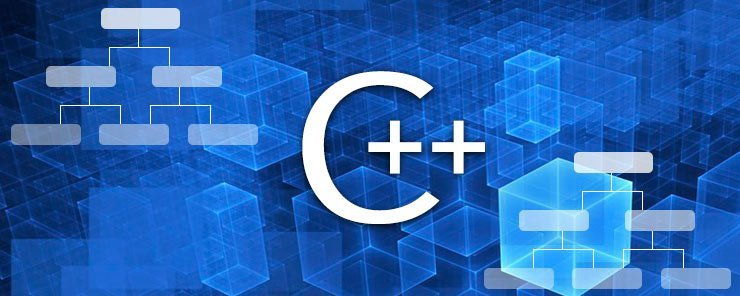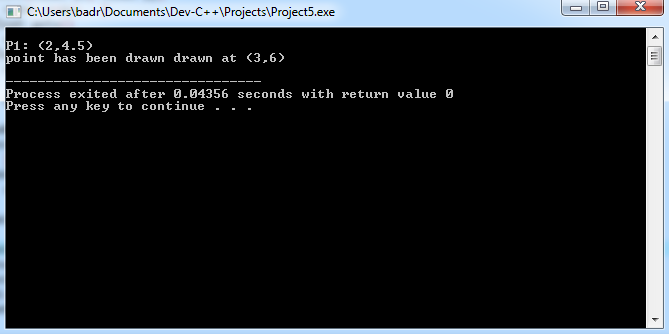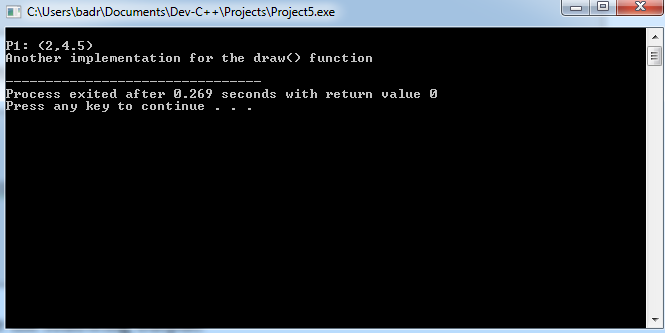# Learn About Inheritance in C++ Programming

0
2234Back again to OOP. This time, we are going to tackle an advanced OOP topic: Inheritance. So, grab your coffee, relax in your favorite chair, and give me your attention.

What is Inheritance?
The term inheritance means to utilize an existing (parent or base) class to create a new (child or derived) class that inherits the parent’s attributes and functions, and extends them by adding its own ones.

Why Inheritance?
Inheritance increases code sharing, and reusability. This gives you two important benefits:

First: it saves time, because you don’t need to reinvent the wheel. Second: it enriches the developing process as a result of ideas and efforts sharing.

Syntax

```class CHILD : ACCESSMODE PARENT
```

Example
Starting with the point class that we have previously defined, we will define a new class that will inherit and extend its features. Read the following program:

```#include <iostream>
using namespace std;
class point
{
protected:
float x,y;
public:
point(float px, float py)
{ x=px; y=py;	}
float getx()
{ return x; }
float gety()
{ return y; }
};
class point2 : public point
{
public:
point2(float x,float y) : point(x,y)
{ }
void draw()
{ cout << "point has been drawn drawn at (" << x << "," << y << ")\n"; }
};
int main()
{
point2 p1(2,4.5);
cout << "\nP1: (" << p1.getx() << "," << p1.gety() << ")\n";
point2 p2(3,6);
p2.draw();
return 0;
}
```

Let’s see how it will behave when executed:Okay, now onto the explanation.

• First, the parent (base) class point is defined:
• The two member variables x and y are marked protected. Being protected makes member attributes and functions accessible only inside the base class, and from any class derived from it.
• The constructor, getx, and gety functions are made public as usual.

Note
For member variables in a base class to be accessible from derived classes inheriting it, they need to be made protected.

• Next, the child (derived) class point2 is defined:
`class point2 : public point`

This declares the class point2 as a subclass of the class point.

• The derived class defines its own public constructor:
```point2(float x,float y) : point(x,y)
{ }```

This constructor does nothing but call its parent’s constructor.

• Now, the child class is going to put its own mark; defines the draw member function:
```void draw()
{ cout << "point has been drawn drawn at (" << x << "," << y << ")\n"; }
```

Remember
public: accessible from everywhere.
private: accessible only from it own class.
protected: accessible from its own class, and from any derived child class.

Overriding Parent’s Functions
In the above example, the point2 class has used the member functions of its parent unchanged and added one of its own. The derived class could define its own implementation (version) of (some or all) member functions defined in the base class. A member function defined in a derived class overrides the function with the same name as its parent.

Let’s take inheritance in the point example to another level by defining class point3 that is derived from point2, and override the draw() function.

In addition to the code above, here is the definition of point3:

```class point3 : public point2
{
public:
point3(float x,float y) : point2(x,y)
{ }
void draw()
{
cout << "Another implementation for the draw() function\n";
}
};
```

And the modified main() function:

```int main()
{
point3 p1(2,4.5);
cout << "\nP1: (" << p1.getx() << "," << p1.gety() << ")\n";
point3 p2(3,6);
p2.draw();
return 0;
}
```

Executing this code should give the following output:Calling the draw() function from objects of the point3 class will cause the point3 version of the function to be called (not that of its parent).

Abstract Classes
An abstract class is a class that can’t be instantiated. One may ask, why should we even create such type of classes if we can’t use it to create objects. The answer is clear: it acts as a “generic” base to derive other classes from.

For a class to be considered abstract, it should contain one or more pure virtual functions.

– Virtual Function?!

Yes, this is the term I need you to remember until we talk about this topic in the next article: Abstract Classes and Virtual Functions.

Summary